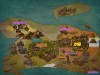# 星星灰暗着。# 星星灰暗着。

### 贪心与DP记录/计算几何能做的

posted on 2019-02-20 09:21:21 | under Diary |

## $[HNOI2018]$ 排列&[集训队作业2018]三角形

$$V_{ab}=\sum_{j=1}^{n_a}(i+j)w_{a_j}+\sum_{j=1}^{n_b}(i+j+n_a)w_{b_j}$$ $$V_{ba}=\sum_{j=1}^{n_b}(i+j)w_{b_j}+\sum_{j=1}^{n_a}(i+j+n_b)w_{a_j}$$ 那么 $V_{ab}-V_{ba}=n_aW_b-n_bW_a$ 。

## $[JSOI2018]$ 部落战争

$70pts$ 大概是运用解析几何的知识求下每个横纵坐标的 $A$ 凸包边界，或者直接 $O(\log n)$ 判一下是否在凸包内，复杂度是 $O(qn\log n)$ 的。
$100pts$ 其实我并不知道为什么可以直接这样转换。